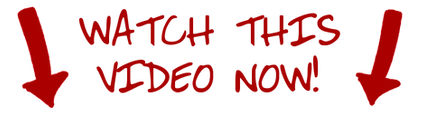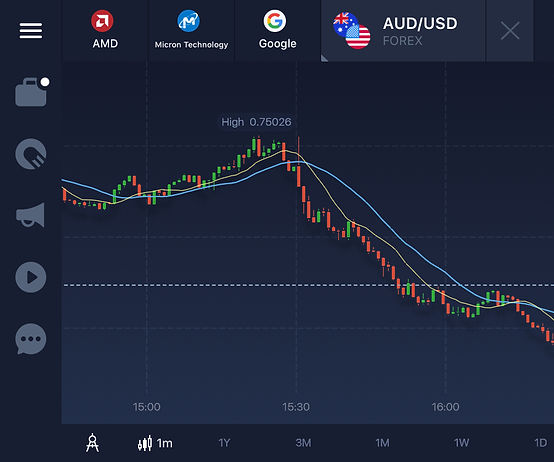# what are moving averages ?

The Moving Average is amongst the most popular of technical analysis tools. It's used to gauge the general direction of the current trend, and is based on past prices.

There are four types of Moving Average, namely;

1. Simple Moving Average (SMA)

2. Weighted Moving Average (WMA)

3. Exponential Moving Average (EMA)

4. Smoothed Simple Moving Average (SSMA)

Watch this short video that will help you understand it better. It will take less than a minute of your time.The two most widely used of the four are the SMA & the EMA. The SMA gives us the average asset price over a period of time.. It can be 3 days, 10 days, or even 20 weeks. It's up to the trader.

## Interpreting the Moving average

The Moving Average is used to determine the trend. One of the simplest ways of doing this to plot one MA on the chart. In this case we will use the SMA (Simple Moving Average). If the price (the candlesticks/line) is below the MA, then we have a bearish trend (downward). Conversely, if the SMA is below the chart, this is indicative of a bullish trend (upward).The image above shows an upward trend of the USD/ZAR (United States Dollar vs the South African Rand). Although not perfect, you can see that the price tends to remain above the SMA 10 line. Towards the end, there's a trend reversal, and the SMA goes above the price, signalling a downtrend.Now, using just one SMA can sometimes be a little risky, so what some traders do is plot two MA's. one faster than the other. For example, you plot one 10 SMA, and you make the other one a 20 SMA. In the image above you can see two moving averages. The faster MA (blue SMA-20) must always be above the slower (yellow SMA-10) MA.

The image immediately above also shows what's known as a crossover. At around 15:30 (bottom scale) the slower MA (yellow) crosses the blue line, and goes beneath the faster blue one, and you get a nice bearish trend.

### Moving average as support & resistance

The MA can also be used as dynamic support and resistance levels. When the price touches, or almost touches the MA from above, you place a CALL option. When it does so from below, you PUT. The image below of the USD/SGD (UD Dollar vs Singapore Dollar) demonstrates this.